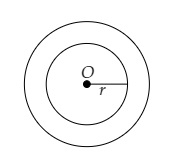# The area enclosed between the concentric circles is $770\ cm^2$. If the radius of the outer circle is $21\ cm$, find the radius of the inner circle.

Given:

The area enclosed between the concentric circles is $770\ cm^2$.

The radius of the outer circle is $21\ cm$.

To do:

We have to find the radius of the inner circle.

Solution:

Let $r$ be the radius of the inner circle.Area of a circle of radius $r=\pi r^2$

According to the question,

$\pi (21)^{2}-\pi r^{2}=770$

$\frac{22}{7}(21)^{2}-\frac{22}{7} r^{2}=770$

$\frac{22}{7} \times 441-\frac{22}{7} r^{2}=770$

$\Rightarrow 1386-\frac{22}{7} r^{2}=770$

$\Rightarrow \frac{22}{7} r^{2}=1386-770$

$\Rightarrow \frac{22}{7} r^{2}=616$

$\Rightarrow r^{2}=\frac{616 \times 7}{22}$

$\Rightarrow r^{2}=28 \times 7$

$\Rightarrow r^{2}=196$

$\Rightarrow r^{2}=(14)^{2}$

$\Rightarrow r=14\ cm$

The radius of the inner circle is $14\ cm$.

Updated on: 10-Oct-2022

27 Views